# Test: Networks- 2

Test Description

## 20 Questions MCQ Test GATE Electrical Engineering (EE) 2023 Mock Test Series | Test: Networks- 2

Test: Networks- 2 for Electronics and Communication Engineering (ECE) 2023 is part of GATE Electrical Engineering (EE) 2023 Mock Test Series preparation. The Test: Networks- 2 questions and answers have been prepared according to the Electronics and Communication Engineering (ECE) exam syllabus.The Test: Networks- 2 MCQs are made for Electronics and Communication Engineering (ECE) 2023 Exam. Find important definitions, questions, notes, meanings, examples, exercises, MCQs and online tests for Test: Networks- 2 below.
Solutions of Test: Networks- 2 questions in English are available as part of our GATE Electrical Engineering (EE) 2023 Mock Test Series for Electronics and Communication Engineering (ECE) & Test: Networks- 2 solutions in Hindi for GATE Electrical Engineering (EE) 2023 Mock Test Series course. Download more important topics, notes, lectures and mock test series for Electronics and Communication Engineering (ECE) Exam by signing up for free. Attempt Test: Networks- 2 | 20 questions in 60 minutes | Mock test for Electronics and Communication Engineering (ECE) preparation | Free important questions MCQ to study GATE Electrical Engineering (EE) 2023 Mock Test Series for Electronics and Communication Engineering (ECE) Exam | Download free PDF with solutions
 1 Crore+ students have signed up on EduRev. Have you?
Test: Networks- 2 - Question 1

### The Y parameter for total network is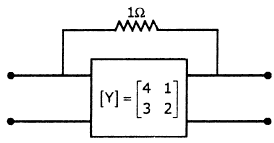Detailed Solution for Test: Networks- 2 - Question 1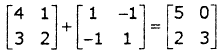There are two networks connected in parallel. Thus there Y-parameter will be added.

*Answer can only contain numeric values
Test: Networks- 2 - Question 2

###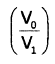in the circuit shown below is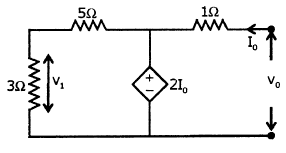Detailed Solution for Test: Networks- 2 - Question 2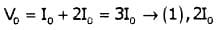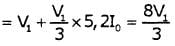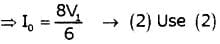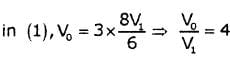Test: Networks- 2 - Question 3

### The value of zin for the circuit shown below is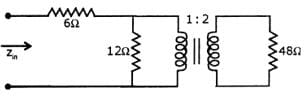Detailed Solution for Test: Networks- 2 - Question 3

Net impedance on primary side of transformer is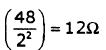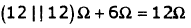*Answer can only contain numeric values
Test: Networks- 2 - Question 4

Equivalent resistance across AB is _____Ω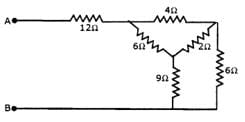Detailed Solution for Test: Networks- 2 - Question 4

Convert Δ to Y and then use series parallel combination

Test: Networks- 2 - Question 5

In the circuit given below v,n = u(—t) + u(t) V

Then vc (t) for t > 0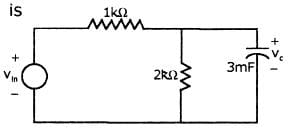Detailed Solution for Test: Networks- 2 - Question 5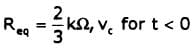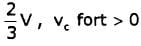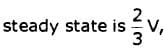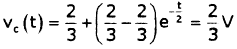Test: Networks- 2 - Question 6

A voltage V = 12√2 cos 5000t V is applied to the circuit shown below.

The current i(t) is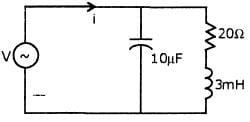Detailed Solution for Test: Networks- 2 - Question 6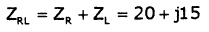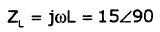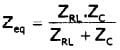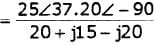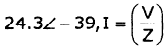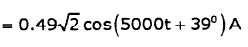Test: Networks- 2 - Question 7

In the circuit shown below, the voltage source has been connected for a long time enough for steady state condition to be reached. At time t = 0, switch S is opened. Then open circuit voltage across AB is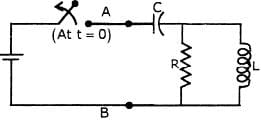Test: Networks- 2 - Question 8

Calculate Vx & Ix in the circuit shown below.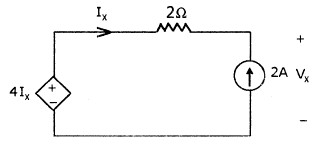Detailed Solution for Test: Networks- 2 - Question 8

Ix = -2A
Apply KCL
-4Ix + 2Ix + Vx = 0
Vx = 4Ix -2Ix = 2Ix = -4V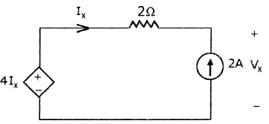Test: Networks- 2 - Question 9

Determine the value of I' in the circuit shown below.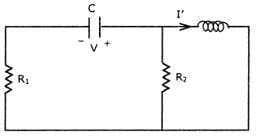Test: Networks- 2 - Question 10

Determine RMS values of voltage across capacitor (0.25F), current throughcapacitor, if voltage across capacitor is 4 + 4 cos (9t) - 5cos (4t)

Detailed Solution for Test: Networks- 2 - Question 10

Vc = 4 + 4 cos(9t) - 5cos(4t)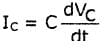= 1/4 {-4(9) sin(9t) + 5(4) sin (4t)}
⇒ -9 sin(9t) + 5 sin (4t)

RMS value of VL is =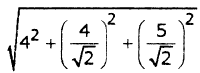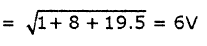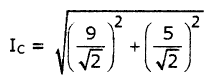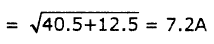*Answer can only contain numeric values
Test: Networks- 2 - Question 11

The value of V, in the circuit shown below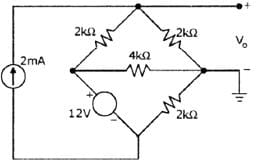Detailed Solution for Test: Networks- 2 - Question 11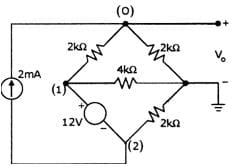V1— V2 =12V................. (1)

At node 0: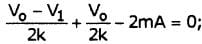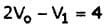--------(2)

At supernode 1 - 2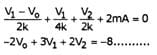(3)

Solving 1, 2 & 3; Vo = 4.5V; V1 = 5V; V2 = —7V

Test: Networks- 2 - Question 12

For the circuit shown in figure, find V(t) when Vs = 2sin(500t) V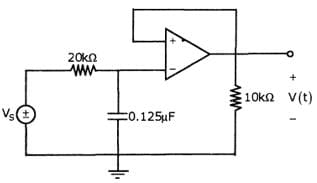*Answer can only contain numeric values
Test: Networks- 2 - Question 13

In the circuit shown below, the switch is closed at time t=0. The steady state value of the voltage Vc is_____ V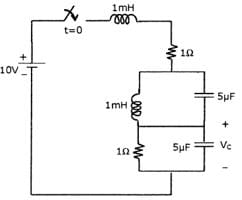Detailed Solution for Test: Networks- 2 - Question 13

At steady state inductor short circuit, capacitor is open circuit. Thus voltage Vc is equal to voltage across 1 ohm resistance.

Test: Networks- 2 - Question 14

Which of the following statements are true for the circuit shown below?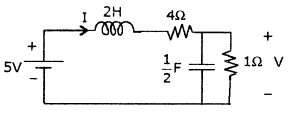1. It is first order with steady state value of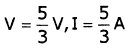2. It is second order with steady state value of  V = iV, I = 1A
3. The network function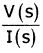has one pole I(s)
4. The network functionhas two poles I(s)

Choose the correct one

Detailed Solution for Test: Networks- 2 - Question 14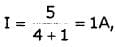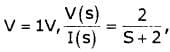Where V(t) is the voltage across the capacitor

This has a pole at S = -2.

Test: Networks- 2 - Question 15

In the circuit shown below, it is found that the input ac voltage and current are in phase. The value of coupling coefficient K and the dot polarity of the coil PQ are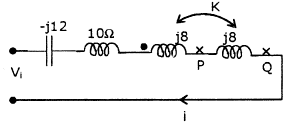Detailed Solution for Test: Networks- 2 - Question 15

If input ac voltage and current are in phase then net impedance is equal to zero. Net inductive impedance = Net capacitive impedance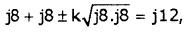, Thus k = 21 and dot must be at Q so that net inductance =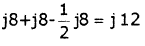Test: Networks- 2 - Question 16

For the given circuit, switch closes at t = 0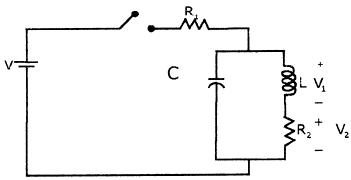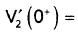Detailed Solution for Test: Networks- 2 - Question 16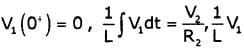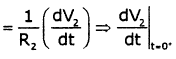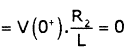Test: Networks- 2 - Question 17

The voltage applied to a particular circuit comprising two components connected in series is given by:

V = (30 + 40 sin 103t +25sin2x103t +15sin4x103t) Vand the resulting current is given by

= 0.743 sin (103t + 1.190) + 0.781sin(2x103t + 0.896) + 0.636sin(4x103t + 0.559)A

Q. Average power supplied is

Detailed Solution for Test: Networks- 2 - Question 17

Average Power P is given by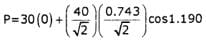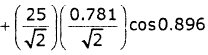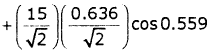P = 0 + 5.523+ 6.099+ 4.044 =15.67W

Test: Networks- 2 - Question 18

The voltage applied to a particular circuit comprising two components connected in series is given by:

V = (30 + 40 sin103t +25sin2x103t +15sin4x103t) Vand the resulting current is given by

= 0.743 sin (103t + 1.190) + 0.781sin(2x103t + 0.896) + 0.636sin(4x103t + 0.559)A

Q. Which of the following element is not a part of the circuit?

Detailed Solution for Test: Networks- 2 - Question 18

The expression for the voltage contains a dc component of 30V. However there is no corresponding term in the expression for current. This indicates that one of the components is a capacitor (since in a dc circuit a capacitor offers infinite impedance to a direct current). Since power is delivered to the circuit the other component is a resistor.

Test: Networks- 2 - Question 19

Consider the circuit shown in figure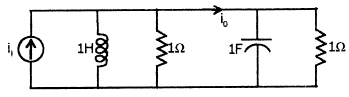Q.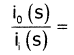Detailed Solution for Test: Networks- 2 - Question 19

Using current division rule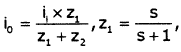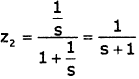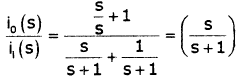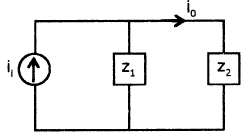Test: Networks- 2 - Question 20

Consider the circuit shown in figureQ. If input current is δ(t), then io (t) (in Amps) will be

Detailed Solution for Test: Networks- 2 - Question 20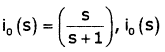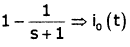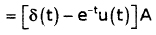## GATE Electrical Engineering (EE) 2023 Mock Test Series

22 docs|274 tests
 Use Code STAYHOME200 and get INR 200 additional OFF Use Coupon Code
Information about Test: Networks- 2 Page
In this test you can find the Exam questions for Test: Networks- 2 solved & explained in the simplest way possible. Besides giving Questions and answers for Test: Networks- 2, EduRev gives you an ample number of Online tests for practice

## GATE Electrical Engineering (EE) 2023 Mock Test Series

22 docs|274 tests